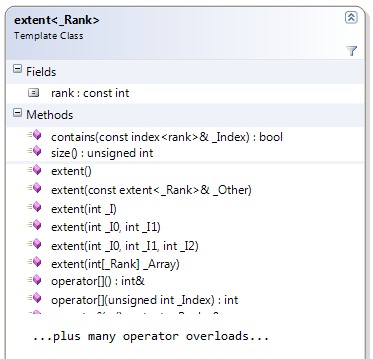## concurrency::extent from amp.h

Mon, September 5, 2011, 06:23 PM under GPGPU | ParallelComputing

#### Overview

We saw in a previous post how index<N> represents a point in N-dimensional space and in this post we'll see how to define the N-dimensional space itself.With C++ AMP, an N-dimensional space can be specified with the template class extent<N> where you define the size of each dimension.

From a look and feel perspective, you'd expect the programmatic interface of a point type and size type to be similar (even though the concepts are different). Indeed, exactly like index<N>, extent<N> is essentially a coordinate vector of N integers ordered from most- to least- significant, BUT each integer represents the size for that dimension (and hence cannot be negative).

So, if you read the description of index, you won't be surprised with the below description of extent<N>

• There is the rank field returning the value of N you passed as the template parameter.
• You can construct one extent from another (via the copy constructor or the assignment operator), you can construct it by passing an integer array, or via convenience constructor overloads for 1- 2- and 3- dimension extents. Note that the parameterless constructor creates an extent of the specified rank with all bounds initialized to 0.
• You can access the components of the extent through the subscript operator (passing it an integer).
• You can perform some arithmetic operations between extent objects through operator overloading, i.e. ==, !=, +=, -=, +, -.
• There are operator overloads so that you can perform operations between an extent and an integer: -- (pre- and post- decrement), ++ (pre- and post- increment), %=, *=, /=, +=, –= and, finally, there are additional overloads for plus and minus (+,-) between extent<N> and index<N> objects, returning a new extent object as the result.

In addition to the usual suspects, extent offers a contains function that tests if an index is within the bounds of the extent (assuming an origin of zero). It also has a size function that returns the total linear size of this extent<N> in units of elements.

#### Example code

```  extent<2> e(3, 4);
_ASSERT(e.rank == 2);
_ASSERT(e.size() == 3 * 4);
e += 3;
e += 6;
e = e + index<2>(3,-4);
_ASSERT(e == extent<2>(9, 9));
_ASSERT( e.contains(index<2>(8, 8)));
_ASSERT(!e.contains(index<2>(8, 9)));```

#### Usage

The extent class on its own simply defines the size of the N-dimensional space. We'll see in future posts that when you create containers (arrays) and wrappers (array_views) for your data, it is an extent<N> object that you'll need to use to create those (and use an index<N> object to index into them). We'll also see that it is a extent<N> object that you pass to the new parallel_for_each function that I'll cover in the next post.